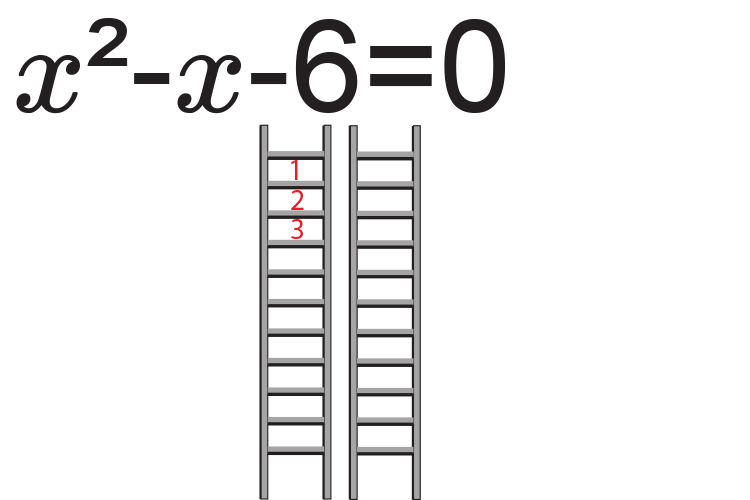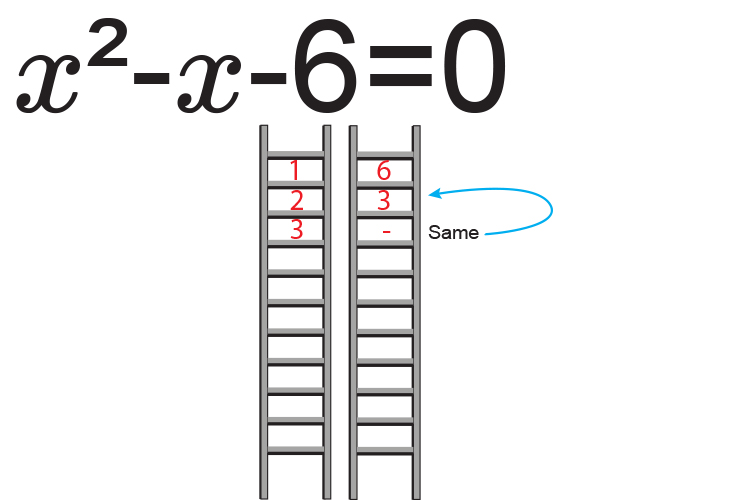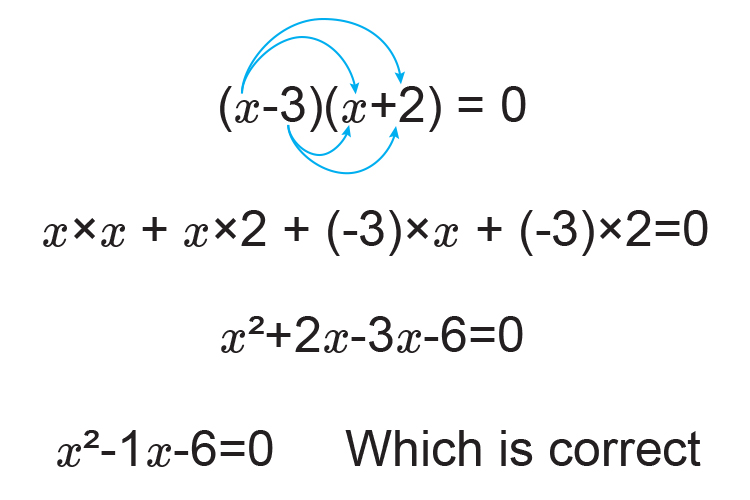# Factorising quadratics - (easy) Example 3

Factorise the quadratic x^2-x-6=0The factors of -6  are:

1 and 6

2 and 3

Now ask yourself what factors of c equal b.

i.e. What factors of -6 add to give -1

-3+2=-1   and  -3times2=-6

Therefore:

x^2-x-6=0

Can be written as:

(x-3)(x+2)=0

Check by multiplying outThe roots of x^2-x-6=0

are (x-3)=0

and (x+2)=0

Now solve for x

x=3   or  x=-2

The roots of x^2-x-6=0  are x=3  or x=-2